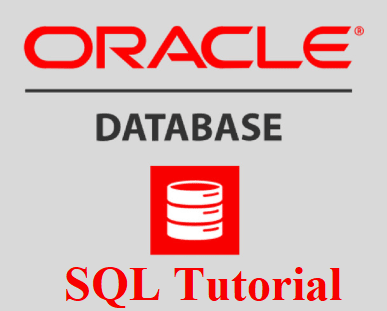# COUNT(), AVG() and SUM() Functions in Oracle SQL | Oracle SQL Tutorials -14

Hi,

I will explain COUNT(), AVG() and SUM() Functions in Oracle SQL in this post of Oracle SQL Tutorial series.Read the previous post of this tutorial series before this.

MIN() and MAX() Functions in Oracle SQL | Oracle SQL Tutorials -13

The COUNT() function

You can find the number of rows according to a specified criterion using the COUNT Function.

COUNT() Syntax

COUNT() Function syntax is as follows.

```

SELECT COUNT(*) FROM table_name;

SELECT COUNT(column_name) FROM table_name;

SELECT COUNT(column_name) FROM table_name WHERE condition;```
COUNT Function examples are as follows.
```SQL> select count(*) from hr.employees;

COUNT(*)
----------
106

SQL> select count(first_name) from hr.employees;

COUNT(FIRST_NAME)
-----------------
106

SQL> select count(distinct salary) from hr.employees;

COUNT(DISTINCTSALARY)
---------------------
57

SQL> select count(*) from hr.employees where salary>10000;

COUNT(*)
----------
22

SQL>```

The AVG() function

You can find the average value of a numeric column using the AVG Function.

AVG () Syntax

AVG () Function syntax is as follows.

```SELECT AVG(column_name) FROM table_name WHERE condition;

```
AVG Function examples are as follows.

```SQL> select avg(salary) from hr.employees;

AVG(SALARY)
-----------
7390.71698

SQL>
SQL> select avg(salary) from hr.employees  where salary>5000;

AVG(SALARY)
-----------
9758.98413

SQL>

```

The SUM () function

You can find the total sum of a numeric column using the SUM Function.

SUM () Syntax

SUM () Function syntax is as follows.

```SELECT SUM(column_name) FROM table_name WHERE condition;
```
SUM Function examples are as follows.
```SQL> select sum(salary) from hr.employees;

SUM(SALARY)
-----------
783416

SQL>
SQL> select sum(salary) from hr.employees where salary>5000;

SUM(SALARY)
-----------
614816

SQL>```
```

```

#### Do you want to learn Oracle Database for Beginners, then read the following articles.

Oracle Tutorial | Oracle Database Tutorials for Beginners ( Junior Oracle DBA )